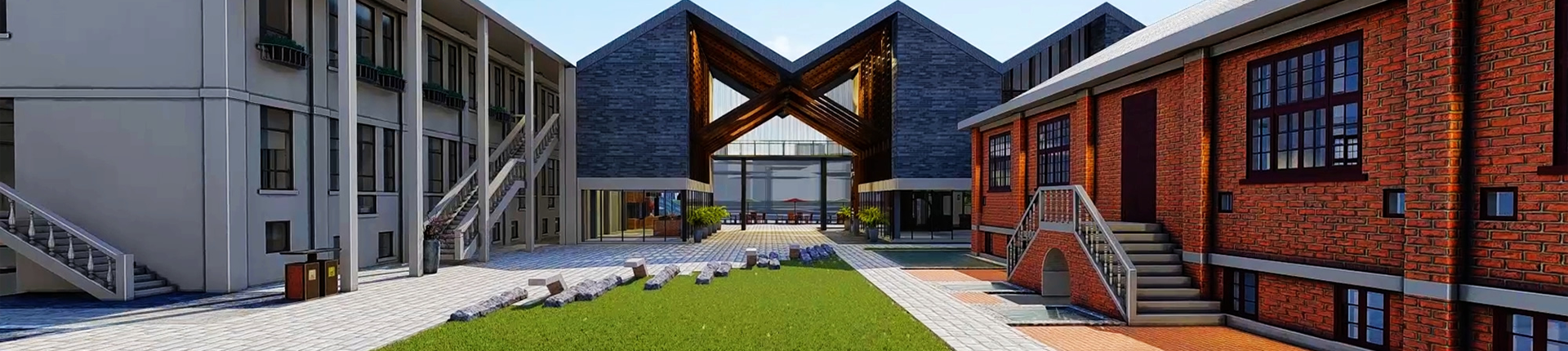ENGLISH# The calculation rules for the quantity of vertical transportation engineering

2021-04-08
announcer:
View: 7

1. General rules for calculating the vertical transportation volume of buildings: Buildings are calculated according to the construction area. Including the calculation of the area of the building area and the area of the equipment pipeline layer with a height of 2.2m.

2. Vertical transportation of buildings (1~6 floors): When the number of floors of a building is 6 floors or less or the eaves height is less than 20m, the calculation is based on the building area of 6 floors or less. Including construction area such as basement and roof stairwell.

3. Vertical transportation of high-rise buildings and additional fees:

1. Any building with 6 floors or more or the eaves height of more than 20m can be counted for vertical transportation and additional fees. When the height is more than 20m, the difference between the eaves height of the building and 20m is divided by 3.3m (the remainder is not counted) ) Is the number of floors (except paragraphs 5 and 6), and the cumulative construction area is calculated.

2. When the area of the upper floor is less than 50% of the area of the lower floor, the calculation shall be divided into two parts vertically. The high range of the number of floors (or eaves height) and the low range of the number of floors (or eaves) are calculated according to the rule of 1 paragraph respectively.

3. When the area of the upper floor is greater than or equal to 50% of the floor area of the lower floor, the number of floors shall be calculated according to paragraph 1, and multiplied by the arithmetic average of the actual floor area of the building with a height of 20m or more to calculate the engineering quantity.

4. When the eaves height of the building is less than 20m and the number of floors is above 6 floors, the 7~8 floors sub-items shall be applied to the building area of 6 floors or more.

5. When the eaves height of the building exceeds 20m, but does not reach 23.3m, regardless of the number of stories, the 7~8 story sub-items shall be applied according to the building area of one floor higher.

6. When the eaves height of the building is more than 28m, but not 29.9m, the building area is calculated according to 3 conversion super high-rise buildings, and the 9-12-story sub-items are applied.

4. For those who apply sub-items of 7 to 8 floors, the remaining construction area should also be applied to sub-items within 6 floors. 5. The podium area of 1 to 6 floors (within 20m) outside the basement and the vertically divided high-rise area shall be applied to the sub-items within 6 floors.